# Quantitative Aptitude Quiz 5Quantitative Aptitude Quiz 5 consists of 10 new pattern questions asked in SBI PO, SBI Clerk, IBPS PO, IBPS Clerk exams. Candidates can practice such new pattern questions to get familiar with the actual type of questions asked in IBPS, SBI, and other insurance exams:

Question 1: In each of the following questions, two equations I and II are given. Solve both the equations and given answer: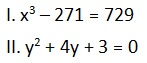a. x is greater than y
b. x is less than equal to y
c. x is less than y
d. x is greater than equal to y
e. x = y or relationship between x and y can’t be determined

a. x is greater than y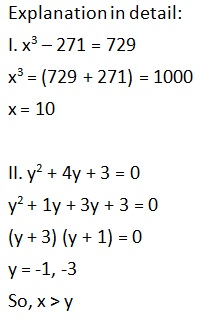Question 2: In each of the following questions, two equations I and II are given. Solve both the equations and given answer: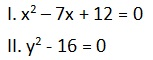a. x is greater than y
b. x is less than equal to y
c. x is less than y
d. x is greater than equal to y
e. x = y or relationship between x and y can’t be determined

e. x = y or relationship between x and y can’t be determined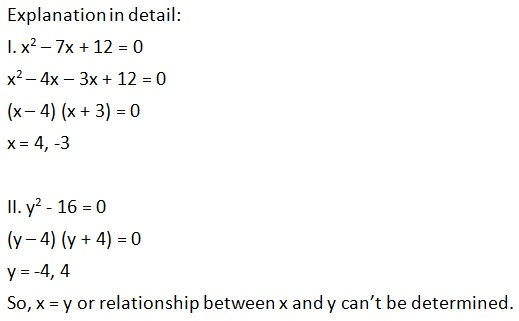Question 3: In each of the following questions, two equations I and II are given. Solve both the equations and given answer: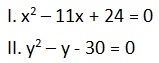a. x is greater than y
b. x is less than equal to y
c. x is less than y
d. x is greater than equal to y
e. x = y or relationship between x and y can’t be determined

e. x = y or relationship between x and y can’t be determined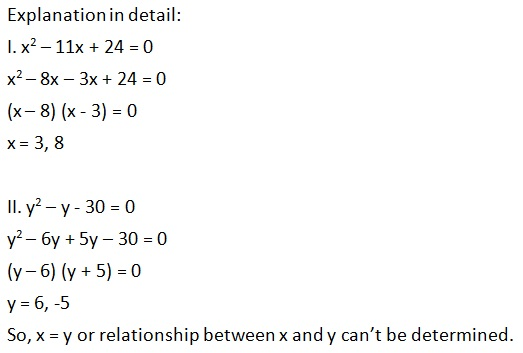Question 4: In each of the following questions, two equations I and II are given. Solve both the equations and given answer: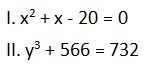a. x is greater than y
b. x is less than equal to y
c. x is less than y
d. x is greater than equal to y
e. x = y or relationship between x and y can’t be determined

c. x is less than y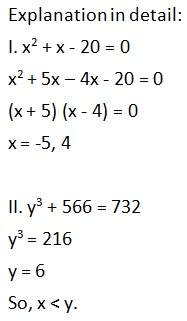Question 5: In each of the following questions, two equations I and II are given. Solve both the equations and given answer: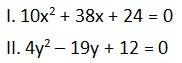a. x is greater than y
b. x is less than equal to y
c. x is less than y
d. x is greater than equal to y
e. x = y or relationship between x and y can’t be determined

c. x is less than y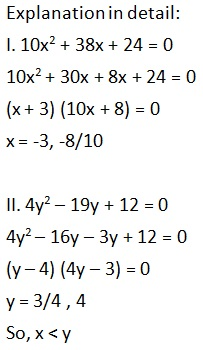Question 6: A 240m long train crosses a platform, which is four times its length, in 9 minutes. What is the speed of the train (m/sec)?

a. 3 m/sec
b. 2.22 m/sec
c. 4.34 m/sec
d. 1.34 m/sec
e. 4 m/sec

b. 2.22 m/sec
Explanation in detail:
Length of the train = 240m
Length of the platform = (4 × 240)m = 960m
Time taken to cross the platform = (9 × 60) seconds = 540 seconds
Speed of the train = (960/540) m/sec = 2.22m/sec
Hence, option b.

Question 7: 6 boys and 5 girls can read a complete book in 3 days while 3 boys and 2 girls can read the same book in 7 days. In how many days 3 boys and 2 girls can read the same book?

a. 7.5 days
b. 9 days
c. 8 days
d. 7 days
e. 6.5 days

d. 7 days
Explanation in detail:
Let 1 day’s work by each boy = x
Let 1 day’s work by each girl = y
6x + 5y = 1/3
3x + 2y = 1/7
So, x = 1/63 and y = 1/21
1 day’s work by 3 boys and 2 girls
= 3(1/63) + 2(1/21)
= 3/63 + 2/21
= 1/7
3 boys and 2 girls can read the same book in 7 days.

Question 8: 568 chocolates are distributed among Sohail, Rupesh and Kamlesh such that Sohail gets 4 times number of chocolates than Rupesh. Rupesh gets 1/3 times number of chocolates than Kamlesh. How many chocolates does Rupesh get and Kamlesh gets ___% less number of chocolates than Sohail.

a. 213, 20%
b. 71, 25%
c. 210, 20%
d. 71, 25%
e. 280, 35%

d. 71, 25%
Explanation in detail:
Let the number of chocolates with Kamlesh = x.
Number of chocolates with Rupesh = x/3
Number of chocoloates with Sohail = 4x/3
x + x/3 + 4x/3 = 568
x = 213
Kamlesh gets 213 chocolates.
Rupesh gets 213/3 = 71 chocolates.
Sohail gets 284 chocolates.
Required percentage = [(284 – 213)/284] × 100
= (71/284) × 100
= 25%

Question 9: Length of a rectangle is 40% more than its Breadth, which is equal to the length of side of a square. Difference between the areas of Rectangle and Square is 90 sqr metre. What is the area of the circle, whose radius is equal to one third of the length of the rectangle?

a. 280 sqr m
b. 135m sqr m
c. 132 sqr m
d. 154 sqr m
e. 183 sqr m

d. 154 sqr m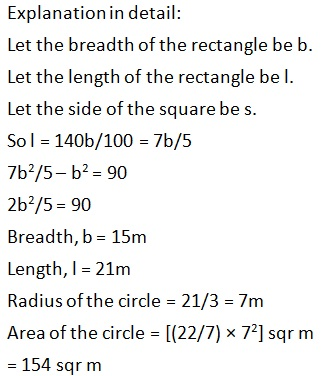Question 10: P, Q and R invested in a business with initial amount of Rs 6500, Rs 8500 and Rs 5500 for 9 months, 3 months and 1 year, respectively. If total profit is Rs 20000, then what is the difference between the profit of P and R?

a. Rs 1000
b. Rs 1500
c. Rs 900
d. Rs 1200
e. Rs 1400

a. Rs 1000
Explanation in detail:
Ratio of investment of P, Q and R = (6500 × 9) : (8500 × 3) : (5500 × 12)
= 39 : 17 : 44
Difference between the profits of P and R
= [(44 – 39)/100] × 20000
= (5/100) × 20000
= Rs 1000

IBPS Home

Back to Home